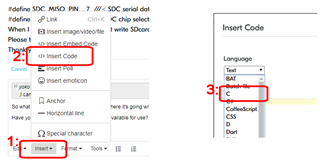# LPC2148 timer0 not working as expected

I am new to ARM and I am trying to produce 1 second delay using timer 0 in LPC2148. But in debug session in Keil, it is giving delay of 4 seconds with 15MHz and 5 seconds with 12 MHz. What is the issue ?

Here is my code.

```#include <lpc214x.h>
int main()
{
IODIR0 = 1; // P0.0 is output pin
IOSET0 = 1; // P0.0 is high
T0PR = 15000000 - 1;
T0TC = T0PC = 0;
T0TCR = 1;  // start
do {
while(T0TC == 0);
T0TC = 0;
IOCLR0 = 1; // P0.0 is low
while(T0TC == 0);
T0TC = 0;
IOSET0 = 1; // P0.0 is high
} while(1);
}```

Parents
• How to properly post source code